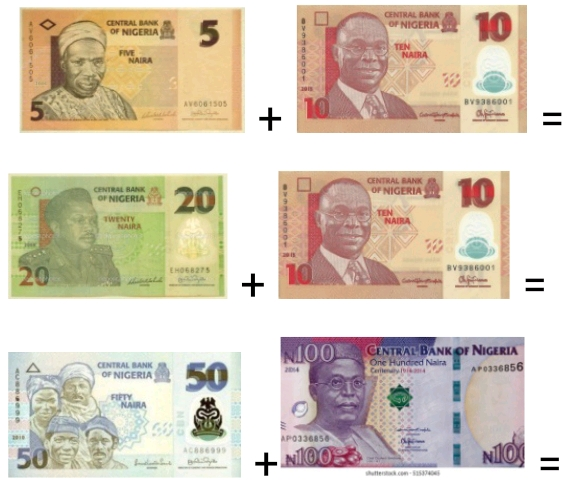# Lesson Notes By Weeks and Term - Nursery 2

Counting/identification of numbers 1 - 900

SUBJECT: Numeracy

TERM: 3rd Term

Week: 7

CLASS – Nursery 2

AGE – 5 years

DURATION – 5 periods of 35 minutes each

TOPIC- Counting/identification of numbers 1-900

CONTENT – Writing 550-600, Number endings. Number spellings 1-120. 10x table. Division, Money problems

INSTRUCTIONAL OBJECTIVES – By the end of the lesson, the pupils should be able to –

1. Count and identify numbers 1-900
2. Write numbers 550-600
3. Write in words 1-120
4. Recite the 10x table
5. Carry out division problems
6. Solve money problems

INSTRUCTIONAL MATERIALS – flash cards, charts, counters

INSTRUCTIONAL TECHNIQUES – Explanation, repetition, questions and answers

ENTRY BEHAVIOUR – The pupils can count numbers 1-800 and spell numbers 1-110

SET INDUCTION –She uses number rhyme to arouse their interest

INSTRUCTIONAL PROCEDURE

PERIOD I: Counting numbers 1-900 and writing 550-600

 STEPS TEACHER’S ACTIVITY PUPILS’ ACTIVITY IIntroduction The teacher reviews the previous lesson introduces the new topic Pupils participate IICounting Using the number chart, she counts number 1-900 repeatedly. Pupils repeat after her IIIWriting numbers She counts and write numbers 550-600 on the board; she also uses flashcards Pupils count and write in their book IVNumber ending She writes the number endings on the board and also uses flashcards Pupils count Evaluation Count and write numbers 550-600 Conclusion She marks their books and corrects them where necessary

PERIOD II& III: Number spellings 1-120. Division. 10x times table

 STEPS TEACHER’S ACTIVITY PUPILS’ ACTIVITY IIntroduction The teacher reviews the previous lesson introduces the new topic Pupils participate IIDrilling She drills them on the spelling of 111-120One hundred and eleven = 111One hundred and twelve = 112One hundred and thirteen =113One hundred and fourteen = 114One hundred and fifteen = 115One hundred and sixteen = 116One hundred and seventeen = 117One hundred and eighteen = 118One hundred and nineteen = 119One hundred and twenty = 120 IIIDivision She explains and writes on the board that division means sharingFor example10 ÷ 2 means sharing 10 things among two people equally.10 ÷ 2 = 5 15 ÷ 3 means sharing 15 things among three people equally15 ÷ 3 = 5 Pupils identify heavy and light objects III10x table She writes the 10x table on the board and recites it severally as the pupils recite after her10 x 1 = 1010 x 2 = 2010 x 3 = 3010 x 4 = 4010 x 5 = 5010 x 6 = 6010 x 7 = 7010 x 8 = 8010 x 9 = 9010 x 10 = 10010 x 11 = 12010 x 12 = 140 Evaluation 1. Dividea. 14 ÷ 2 =b. 9 ÷ 3 =2. Write in words 111-120111=112=113=114=115=116=117=118=119=120=3. Complete the 10x table10 x 9 =10 x 10 =10 x 11=10 x 12= Conclusion She marks their books and corrects them where necessary

PERIOD V: Solving problems with money

 STEPS TEACHER’S ACTIVITY PUPILS’ ACTIVITY IIntroduction The teacher reviews the basic shapes and introduces the new topic Pupils respond IISolving problems with money The teacher solves some money problemsPupils pay attention Evaluation 1. Solve these money problemsConclusion She marks their books and corrects them where necessary